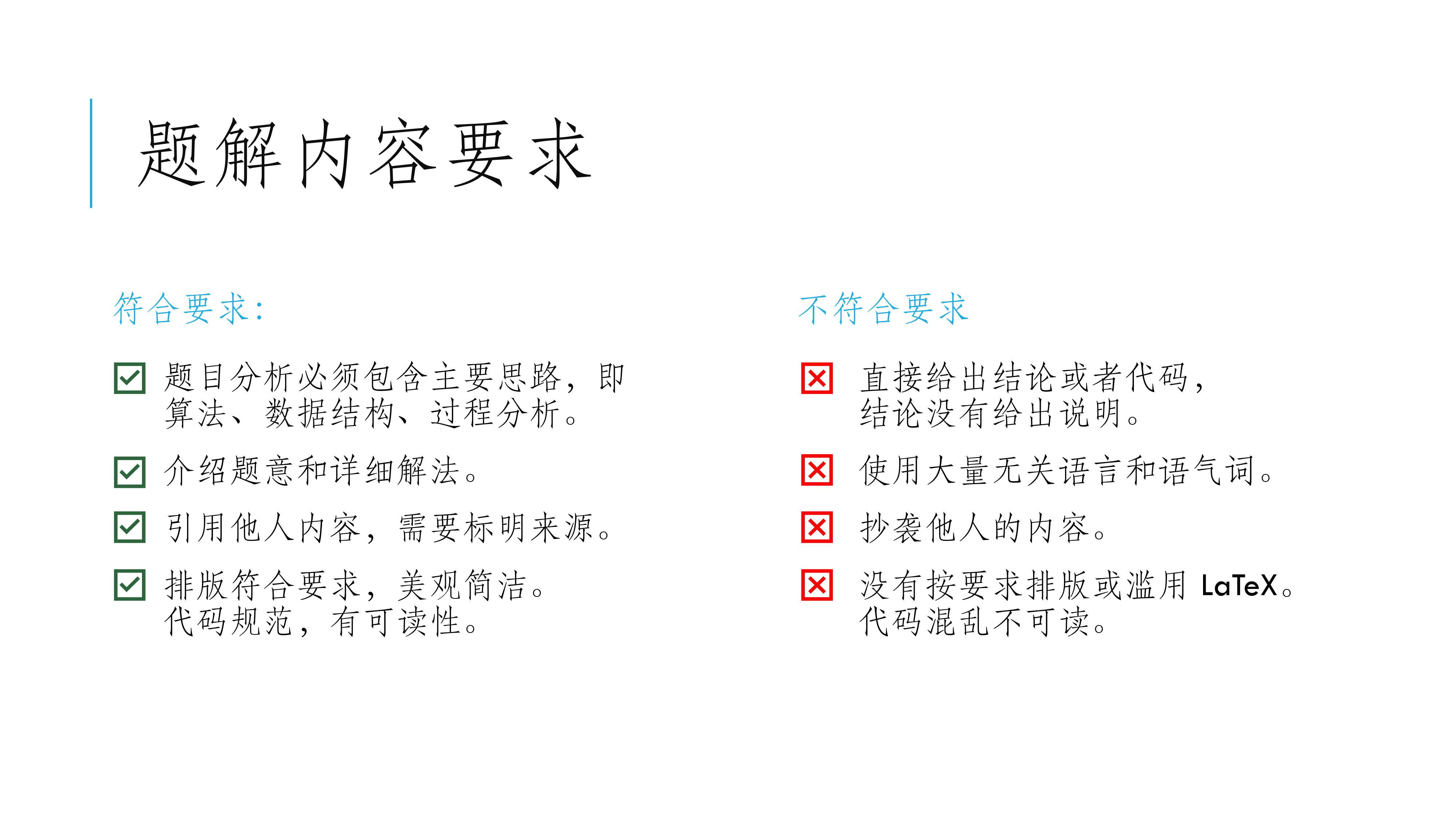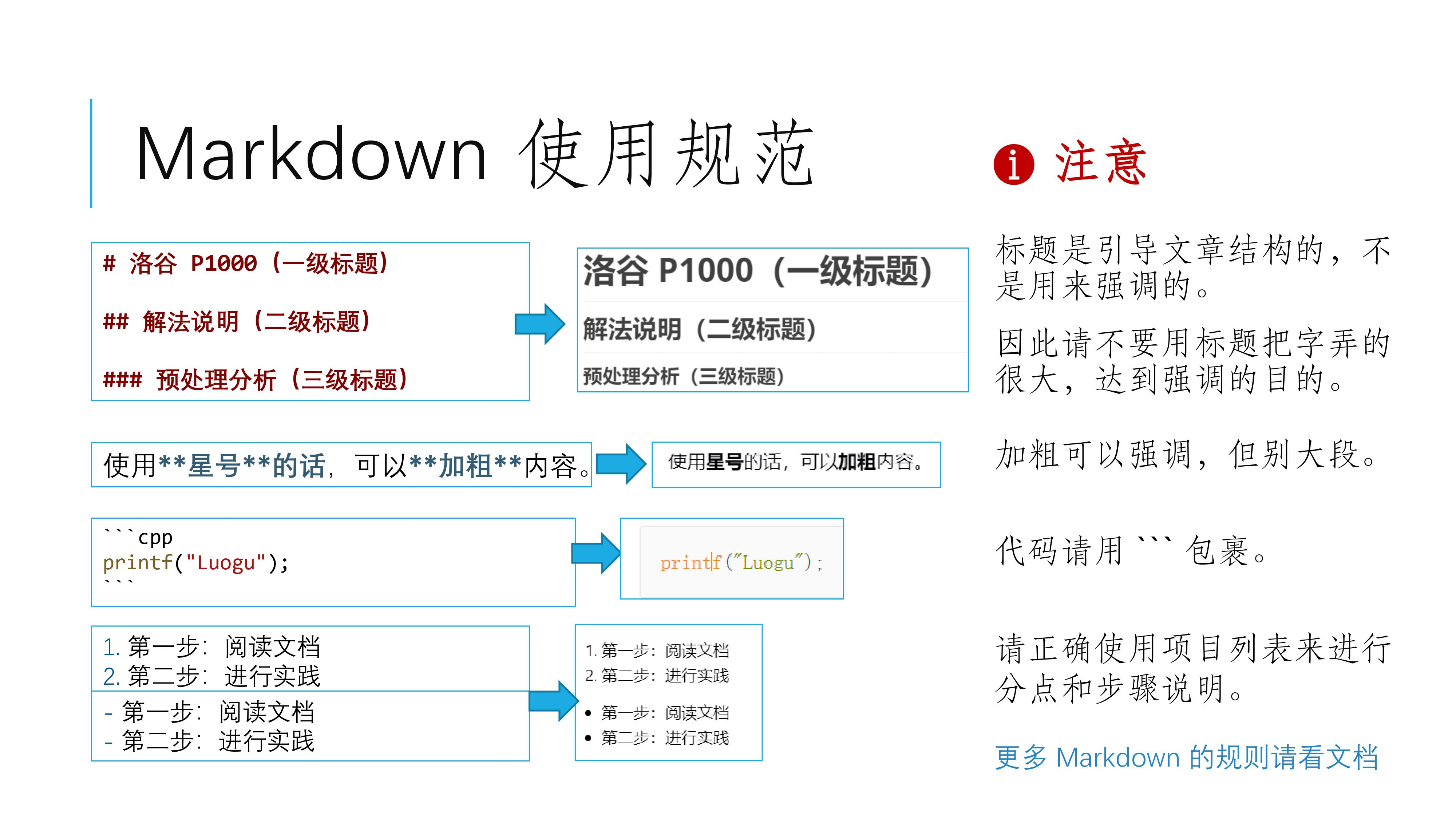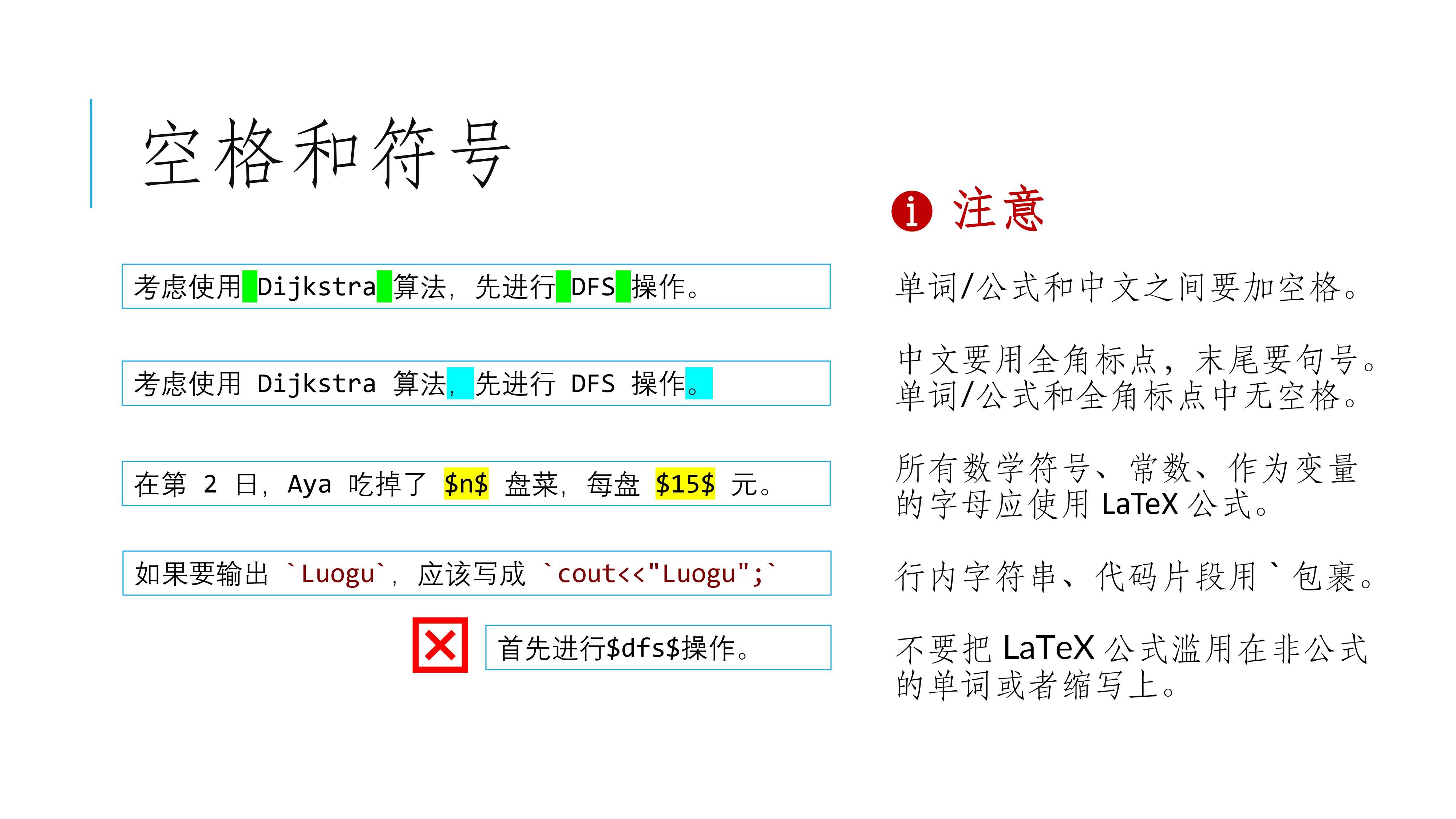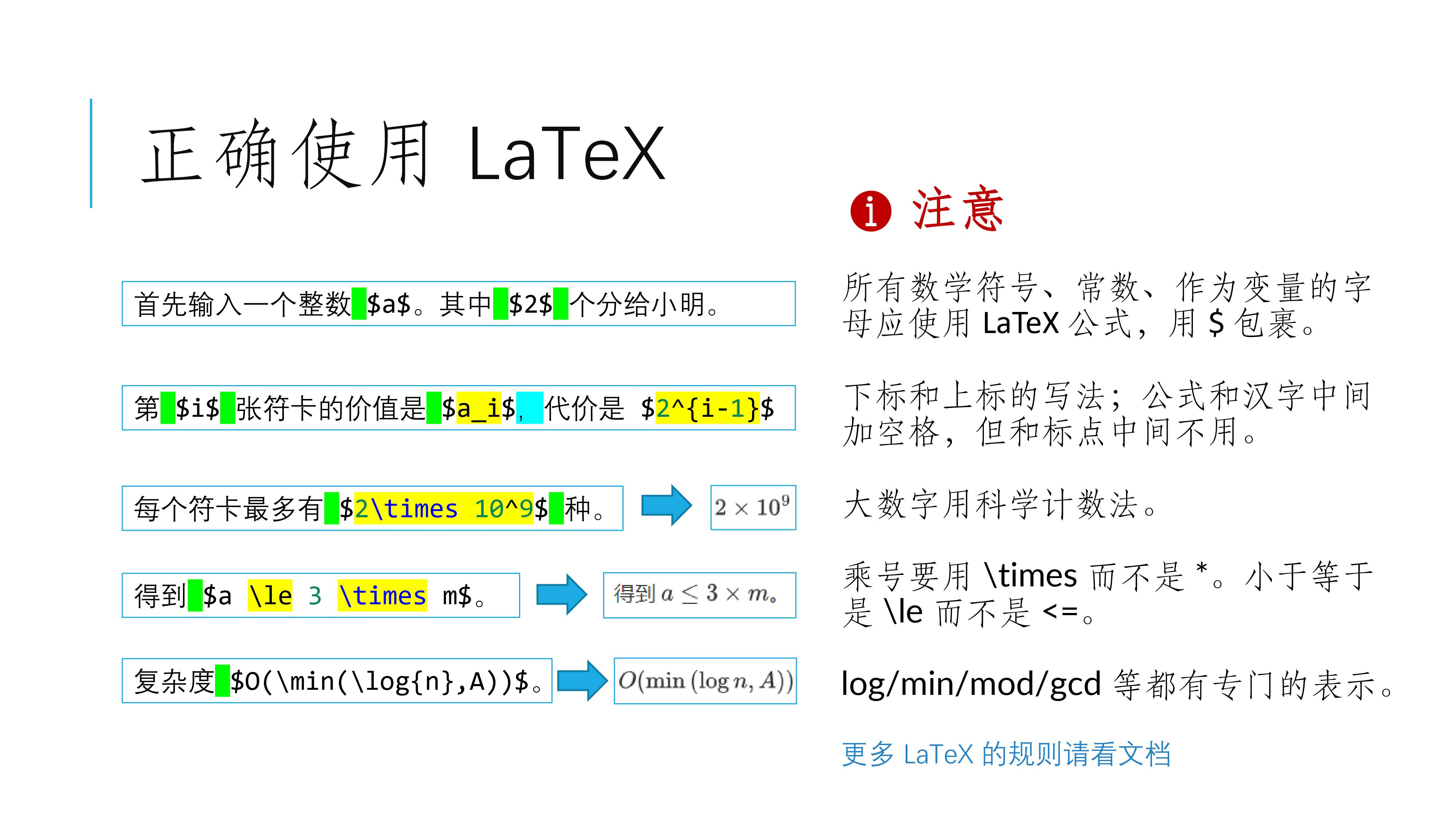# 题解审核及反馈要求

@chen_zhe  Aya 2019-12-07 13:24 回复

#### 图文说明#### 基本规范

• 请正确使用全角中文标点符号。特别地，句末要有句号
• 数学公式（运算式、运算符、参与运算的常数、作为变量的字母等）应使用 LaTeX，非数学公式（一般英文单词、题目名、算法名、人名等）不应使用 LaTeX。
• 中文英文、数字或公式之间以半角空格隔开，但中文标点符号英文、数字或公式之间不应有空格。

#### 题解内容

• 应只包含题目相关内容，包括但不限于题意简述、题目分析等；不应出现大量无关内容，包括但不限于闲聊、吐槽、加戏、求赞、求管理员通过、「蒟蒻的第一篇题解」等内容。
• 对于题面较长的题目，建议加入题意简述，但不应完整复制题面至题解中。
• 题目分析中必须包含做这一道题目的主要思路，包括但不限于：使用了什么算法或数据结构，以及对于相应算法或者数据结构的具体分析。
• 题目分析应给出完整正确的解法与说明，并对解法中的重要结论进行解释与证明。给出的解法应能够在合理的时间复杂度内通过题目。
• 如果需要引用一些来自他人博客的内容，请确保不会侵犯他人的版权，并且必须使用链接标注来源。

#### 排版

• 应使用 markdown 正确排版。
• 应使用 #, ##, ###, #### 符号表示标题行。标题应对文章结构进行引导；不应滥用标题行表示强调与无意义内容。
• 应使用 -, +, * 来表示无序列表，用 1. 来表示有序列表。以下是一个例子：
- QAQ
- QwQ
- QvQ
• QAQ
• QwQ
• QvQ
1. QAQ
2. QwQ
3. QvQ
1. QAQ
2. QwQ
3. QvQ
• 应使用行内代码块表示字符串或代码，如 aabc

• 应使用行间代码块引用代码。

• 应使用 []() 引用链接，如 题解审核及反馈要求[题解审核及反馈要求](https://www.luogu.com.cn/discuss/174934)）。

• 应使用 ![]() 引用图片，如 ![](https://cdn.luogu.com.cn/upload/pic/22071.png)#### 数学公式

• 数学公式（运算式、运算符、参与运算的常数、作为变量的字母等）应使用 LaTeX。同一个数学公式应写在一个 LaTeX 环境内。
• 数学公式中的文本应使用 \text，字符串应使用 \texttt。如 $a \text{ is prime}$（$a \text{ is prime}$），$S = \texttt{aabcd}$（$S = \texttt{aabcd}$）。
• 公式独立成行时应使用行间公式。以下是一个行间公式的例子：
$$a + b = c$$

$$a + b = c$$

• 数学公式中应使用数学语言而非代码语言。
• 赋值语句 a = b; 可以写作 $a \gets b$（$a \gets b$）或 $b \to a$（$b \to a$）。
• 判定语句应使用 $=, \ne, <, \le, >, \ge$（$=, \ne, <, \le, >, \ge$）与艾佛森括号（$[P]$ 当 $P$ 为真时取值为 $1$，$P$ 为假时取值为 $0$）进行描述。
• 整除应使用 $\lfloor \frac{a}{b} \rfloor, \lfloor a / b \rfloor, \lfloor a \div b \rfloor$（$\lfloor \frac{a}{b} \rfloor, \lfloor a / b \rfloor, \lfloor a \div b \rfloor$），不应使用 $\frac{a}{b}$ 或 $a/b$ 直接表示整除。
• 取模应使用 $a \bmod b$（$a \bmod b$） 或 $a \equiv b \pmod p$（$a \equiv b \pmod p$）。
• 不应出现 $a.b$ 等结构体式的写法，如有需要可以使用上下标表示。
• 位运算应使用 $\operatorname{and}, \operatorname{or}, \operatorname{xor}$（$\operatorname{and}, \operatorname{or}, \operatorname{xor}$）。特别地，对于状态压缩 DP 等一类常用位运算实现集合运算的代码，建议在题解中用集合语言直接描述。以下是一个例子：
$$dp_{S \cup \{u\}} \gets dp_{S}+w_u$$

$$dp_{S \cup \{u\}} \gets dp_{S}+w_u$$

• 上下标应使用 $a _ {b} ^ {c}$（$a _ {b} ^ {c}$）进行表示。
• 大数字应使用科学计数法表示，如 $5 \times 10 ^ 9$。
• 时间复杂度的大 $O$ 记号中不应带有常数，如有值域、字符集大小等常量，应使用字母进行表示。
• 应正确使用运算符，如 $+, -, \pm, \times, \cdot, \div, \le, \ge, \mid$（$+, -, \pm, \times, \cdot, \div, \le, \ge, \mid$）。
• 特定的、约定俗成的函数名称应该使用正体，如 $\gcd, \max, \min, \log, \det$（$\gcd, \max, \min, \log, \det$）。特别地，对于一些未定义的函数，应使用 \operatorname，如 $\operatorname{lcm}$（\operatorname{lcm}）。
• 应正确使用大型运算符，如 $\sum, \prod, \bigcup, \bigcap$（$\sum, \prod, \bigcup, \bigcap$）。请注意，大型运算符的优先级较低，例如 $\sum _ {i = 1} ^ n a_i \bmod 998244353$ 表示先将 $a_i$ 对 $998244353$ 取模后再求和；若想表示求和后对 $998244353$ 取模，请使用 $\left(\sum _ {i = 1} ^ n a_i \right) \bmod 998244353$。
• 应正确使用取模符号。
• 取模运算应使用 \bmod，如 $a \bmod b = c$（$a \bmod b = c$）。
• 同余符号应使用 \equiv\pmod，如 $a \equiv c \pmod b$（$a \equiv c \pmod b$）。
• 应正确使用数学结构符号，如 $\frac{a}{b}, \sqrt{a}, \overline{a}, \{a\}$（$\frac{a}{b}, \sqrt{a}, \overline{a}, \{a\}$）。
• 应正确使用箭头符号，用 $\to, \gets$（$\to, \gets$）表示赋值，用 $\Leftarrow, \Rightarrow$（$\Leftarrow, \Rightarrow$）表示因果关系。
• 省略号应使用 $\dots, \cdots, \ldots$（$\dots, \cdots, \ldots$），特别地，矩阵中其它方向的省略号应使用 $\vdots, \ddots$（$\vdots, \ddots$）。
• 波浪线应使用 $\sim$（$\sim$）。
• 连等式应使用 \aligned 环境，分段函数应使用 \cases 环境，矩阵应使用 \bmatrix 环境。以下是一些例子：
\begin{aligned} \sum _ {i = 1} ^ n \sum _ {j = 1} ^ n \gcd(i, j) &= \sum _ {i = 1} ^ n \sum _ {j = 1} ^ n \sum _ {d \mid \gcd(i, j)} \varphi(d) \\ &= \sum _ {d = 1} ^ n \varphi(d) \sum _ {i = 1} ^ n \sum _ {j = 1} ^ n [d \mid \gcd(i, j)] \\ &= \sum _ {d = 1} ^ n \varphi(d) \sum _ {i = 1} ^ n \sum _ {j = 1} ^ n [d \mid i][d \mid j] \\ &= \sum _ {d = 1} ^ n \varphi(d) \left\lfloor \frac{n}{d} \right\rfloor ^ 2 \end{aligned}

\begin{aligned} \sum _ {i = 1} ^ n \sum _ {j = 1} ^ n \gcd(i, j) &= \sum _ {i = 1} ^ n \sum _ {j = 1} ^ n \sum _ {d \mid \gcd(i, j)} \varphi(d) \\ &= \sum _ {d = 1} ^ n \varphi(d) \sum _ {i = 1} ^ n \sum _ {j = 1} ^ n [d \mid \gcd(i, j)] \\ &= \sum _ {d = 1} ^ n \varphi(d) \sum _ {i = 1} ^ n \sum _ {j = 1} ^ n [d \mid i][d \mid j] \\ &= \sum _ {d = 1} ^ n \varphi(d) \left\lfloor \frac{n}{d} \right\rfloor ^ 2 \end{aligned}

$$\lvert 2x - 1 \rvert = \begin{cases} 2x - 1 & x > \frac{1}{2} \\ 0 & x = \frac{1}{2} \\ 1 - 2x & x < \frac{1}{2} \end{cases}$$

$$\lvert 2x - 1 \rvert = \begin{cases} 2x - 1 & x > \frac{1}{2} \\ 0 & x = \frac{1}{2} \\ 1 - 2x & x < \frac{1}{2} \end{cases}$$

$$\begin{bmatrix} f_{i + 1} \\ f_i \end{bmatrix} = \begin{bmatrix} 1 & 1 \\ 1 & 0 \end{bmatrix} \times \begin{bmatrix} f_i \\ f_{i - 1} \end{bmatrix}$$

$$\begin{bmatrix} f_{i + 1} \\ f_i \end{bmatrix} = \begin{bmatrix} 1 & 1 \\ 1 & 0 \end{bmatrix} \times \begin{bmatrix} f_i \\ f_{i - 1} \end{bmatrix}$$

#### 图片与代码

• 题解中引用的图片应简洁、清晰、美观，图片中的文本也需要满足格式要求。请不要引用带有链接的图片。
• 建议题解附有代码，可以在题目分析中穿插给出，也可以在题目分析后完整给出。
• 过长的代码不应放在题解中。如有必要，请使用洛谷云剪贴板。
• 代码应具有一定的可读性，可以适当添加有意义的注释进行阐释。
• 解法不应只在代码注释中描述，应在代码外使用正常文字书写。
• 若需引用他人代码，请请确保不会侵犯他人的版权，并且必须使用链接标注来源，位置建议放于代码之前。
• 不应在代码中加入防抄袭内容。

#### 提交与审核

• 提交审核时应确保题解符合以上规范。
• 若题解被打回，应按照规范与反馈进行修改。多次打回后仍未实质性修改将会封禁博客权限。
• 当一道题的题解数目较多时，管理员会关闭该题的题解提交通道。如果认为自己的题解可以被采纳，可以联系管理员申请加入题解区。申请时需要阐述可以被采纳的理由。
• 如有更多问题，可以进一步询问负责题解审核板块的题目管理志愿者

#### 参考链接

@Gjanuary 2023-04-04 22:42 回复 举报

@CSP_Sept 大佬我写了一段代码测试结果没问题一直显示错误，能帮我看一下嘛？谢谢

#include<iostream>
#include<string.h>
using namespace std;
string tmp;
string result = "";
char op;
int n;
void cz(int index,int i){
int a1=0;
int a2=0;
bool f = true;
for(int i=index;i<tmp.length();i++){
if(tmp[i] !=' ' && f){
a1 = a1*10+(tmp[i]-'0');
}else if(tmp[i] == ' '){
f = false;
}else{
a2 = a2*10+(tmp[i]-'0');
}
}

if(op=='a'){
result += to_string(a1)+"+"+to_string(a2)+"="+to_string(a1+a2)+"\n";
int number = (to_string(a1).length())+(to_string(a2).length())+2+to_string(a1+a2).length();
if(i==n-1){
result += to_string(number);
}else{
result += to_string(number)+"\n";
}

}else if(op=='b'){
result += to_string(a1)+"-"+to_string(a2)+"="+to_string(a1-a2)+"\n";
int number = (to_string(a1).length())+(to_string(a2).length())+2+to_string(a1-a2).length();

if(i==n-1){
result += to_string(number);
}else{
result += to_string(number)+"\n";
}

}else if(op=='c'){
result += to_string(a1)+"*"+to_string(a2)+"="+to_string(a1*a2)+"\n";
int number = (to_string(a1).length())+(to_string(a2).length())+2+to_string(a1*a2).length();
if(i==n-1){

result += to_string(number);
}else{
result += to_string(number)+"\n";
}

}
}

int main(){
cin>>n;
cin.ignore();// 清除缓冲区
for(int i=0;i<n;i++){
getline(cin,tmp);
if('a'<=tmp&&tmp<='c'){
op=tmp;
cz(2,i);
}else{
cz(0,i);
}
}
cout<<result;
return 0;
}
@AC_黄子译21CC 2023-04-08 21:55 回复 举报

1

A->1->C 1

3

A->1->C A->2->B C->1->B A->3->C B->1->A B->2->C A->1->C 7 哪位大佬会啊，需要完整递归题解，谢谢。